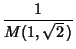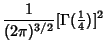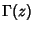## Gauss's Constant

The Reciprocal of the Arithmetic-Geometric Mean of 1 and,(1)(2)(3)(4)

whereis the complete Elliptic Integral of the First Kind andis the Gamma Function.

Finch, S. Favorite Mathematical Constants.'' http://www.mathsoft.com/asolve/constant/gauss/gauss.html